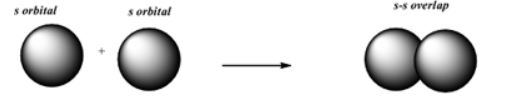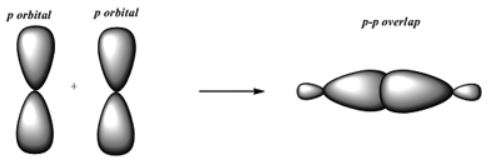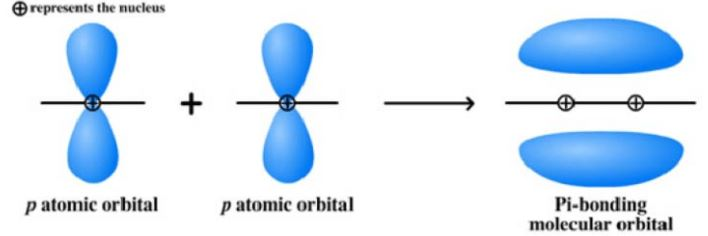# Sigma and Pi Bond

Sigma and pi bond are two types of covalent bonds. If you don’t know what is covalent bond then it is a bond that is formed by mutual sharing of electrons so as to complete their octet or duplet in case of Hydrogen, Lithium and Beryllium.

Depending on the number of electrons the shared number of bonds also vary. If two, four or six electrons are shared, the number of bonds formed will be one, two or three respectively. Thus, covalent bonds are classified into sigma bond and pi bond based on the type of overlapping

## Sigma (𝞂) Bond

This type of covalent bond is formed by the end to end (head-on) overlap of bonding orbitals along the internuclear axis. This is called as head-on overlap or axial overlap. This can be formed by anyone of the following types of combinations of atomic orbitals.

### s-s Overlapping

In this case, there is an overlap of two half-filled s-orbitals along the internuclear axis as shown belows-s Overlap in Sigma Bond

This type of overlapping can be seen in the formation of the H2 molecule. Two Hydrogen atom with a single electron on their s orbital overlap together to form a hydrogen molecule.

### s-p Overlapping

In this case, there is an overlap of half-filled s-orbital of one atom and half-filled p orbital of another atom along the internuclear axis.

This type of overlapping can be seen in the formation of Methane CH4, Ammonia NH3, water H2O

For example, Three p (px,py,pz)orbitals in the carbon atom overlap with the half-filled s orbital of the hydrogen atom.

### p-p Overlapping

This type overlapping takes place between one half-filled p orbital with another half-filled p orbital along the internuclear axisp-p Overlap in Sigma Bonds

This type of overlapping can be seen in the formation of F2 molecules from the Fluorine atoms.

When two Fluorine atoms each containing unpaired electrons with opposite spin each other, then the potential energy of the system decreases.the two p orbitals overlap each other when they acquire minimum potential energy

Note: Helium doesn’t form a diatomic molecule because helium with atomic number 2 has electronic configuration 1S2 and there is no vacant or unpaired orbital.

## Pi (π) Bond

During the formation of Pi bonds, atomic orbitals overlap in such a way that their axes remain parallel to each other and perpendicular to the internuclear axisPi (π) Bonds

During π bond formation atomic orbitals undergo sideways overlapping which gives saucer type charged cloud above and below the internuclear axis.

Note

• All single bonds are 𝞂- bonds
• Multiple bonds contain one 𝞂-bond and the rest are π-bonds.
• π-bond is never formed alone. First, a 𝞂-bond is formed and then the formation of the π- bond takes place. (Exception: C molecule contains both π-bonds)
• A sigma bond is always stronger than pi-bond because the extent of overlapping of atomic orbitals along the internuclear axis is greater than sideways overlapping.
• Electron cloud of 𝞂-bond is symmetrical about the internuclear axis while that of π-bond is not.
• Free relation about a 𝞂-bond is possible but that about π-bond is not.
• The less pi-bonds the more stable the compound is.
• The more the number of Pi-bonds the compound is more reactive.

## Strength of Sigma and Pi Bond

• Strength of a bond basically depends on the extent of overlapping of atomic orbitals.
• Sigma bond form overlapping along the internuclear axis which is powerful than the pi bond which overlaps sideways.
• The area of overlap in pi bonds is lesser as compared to sigma bonds. This the reason why pi-bond breaks first before the sigma bond.
• A pi bond is formed in additional to sigma bond during multiple bond formation.

## Differences Between Sigma and Pi Bond

 Sigma (𝞂)-bond Pi (π)-bond Formed by overlapping along the internuclear axis. It is Formed by the sideways overlapping of the atomic orbitals Powerful bond because overlapping occurs in a larger extent. Less powerful because overlapping occurs in shorter extent. It is the first bond formed during the interaction between atoms. Can’t become the first bond. They are formed later. Exist as a single bond. Single bond existence is not possible. Can’t form multiple bonds. Multiple bond formation. s, p and d orbital can form this bond. Only p and d orbital can form. It decides the shape of the molecule. It decides the length of the molecule. The bond is rotationally symmetric around the internuclear axis. The bond is not rotationally symmetric around the internuclear axis. Present in saturated or unsaturated hydrocarbons. (Example:sp3 tetrahedral) Present only in unsaturated hydrocarbons. (Example:sp2 planar) More reactive. Less reactive.

## Bond Characteristics

### 1. Bond Length

The average distance between the centres of nuclei of bonded atoms is called bond length. It is expressed in terms of picometer (1 pm = 10-12m) or Angstrom (1 Å = 10-10m).

In the covalent compound bond length is the sum of their covalent radii.

Example: Consider an HCl compound, the bond length is d= rH + rCl

In the ionic compound bond length is sum of their ionic radii (d = r+ + r)

#### Factors affecting bond length.

(i) Size of the Atoms.

The bond length increases with increase in the size of the atoms. For example, bond lengths of H-X are in the order

HI> HBr > HCI> HF

(ii) Multiplicity of Bond.

The bond length decreases with the multiplicity of the bond.

(iii) Type of Hybridization

As an s-orbital is smaller in size, greater the s-character shorter is the hybrid orbital and hence shorter is the bond length.

### 2. Bond Energy or Bond Enthalpy

The amount of energy required to break one mole of bonds of a particular type so as to separate them into gaseous atoms is called bond dissociation energy or simply bond energy.

Bond energy is usually expressed in kJmol-1

Further, the greater the bond dissociation energy stronger is the bond

Factors affecting bond energy.

(i) Size of the Atoms

Greater the size of the atoms, greater is the bond length and less is the bond dissociation energy, ie., less is the bond strength.

(ii) Multiplicity of Bonds

For the bond between the same two atoms, greater is the multiplicity of the bond, the greater will be the bond dissociation energy. This is because atoms come closer and secondly, the number of bonds to be broken is more.

(iii) Number of Lone Pair of Electrons Present

Greater the number of lone pairs of electrons present on the bonded atoms greater is the repulsion between the atoms and hence less is the bond dissociation energy.

### 3. Bond Angle

The angle between the lines representing the directions of the bonds, i.e. the orbitals containing the bonding electrons is called the bond angle.

Factor affecting the Bond angle.

(i) Hybridization: The bond angle depends on the central metal atom’s hybridization. Grater the s character, grater the bond angle.

sp – 180o

sp2 – 120o

sp3 – 109o28′

(ii) Repulsion of lone pair of an electron: The presence of lone pair of electron on the central metal atom affects the bond angle. Lone pair on the central metal atom tries to repulse the bond pair which decrease the bond angle.

Example: Bond angle of CH4 is 109whereas the bond angle of NHis 107o because of the presence of one lone pair of electrons.

(iii) Electronegativity: Bond angle decreases with the decreasing in the electronegativity of the central metal atom.

Example: Bond angle of NH3 is 107but the bond angle of PHis 93.50.

## Solved Questions

1. Triple bond in ethyne is formed from……….?

1. Three sigma bonds
2. Three pi bonds
3. One sigma and two pi bonds
4. Two sigma and one pi bond

2. The bond in the formation of Fluorine molecule will be

1. due to PCl5 overlapping
2. due to s-p overlapping
3. due to H2O2>O3>O2
4. due to hybridization

3. In Fluorine molecule formation of p-p orbital take part in formation. The number and type of bonds between two carbon atoms in calcium carbide are (JEE 2005)

1. One sigma, one pi
2. One sigma, two pi
3. Two sigmas, one pi
4. Two sigmas, two pi

4. The number of sigma bonds in P4010 is

1. 6
2. 16
3. 20
4. 7

And: (2)

5. Overlap of which of the following atomic orbitals would be to form the strongest covalent bond.

1. 1s-2s (sigma)
2. 1s-2p (sigma)
3. 2p-2p (pi)
4. 2p-2p (sigma)

6. The angular shape of the ozone molecule consists of

1. 1 sigma and 2 pi bonds
2. 2 sigma and 2 pi bonds
3. 1 sigma and 1 pi bond
4. 2 sigma and one pi bond

7. How is a pi bond formed?

Answer: If a bond between two atoms is broken when one atom is rotated around the bond axis, that bond is called a pi bond. A pi bond is not an axial bond. Pi bonds are formed from the sideways overlap of parallel p orbitals on adjacent atoms. They are not formed from hybrid orbitals.

8. How many sigma and pi bonds are possible

First formed bond will be sigma bond and which only have the independent existence. if the molecule is double bond or triple bond then there is one sigma bond and one or two pi bond respectively.

9. Which is stronger pi or sigma bond?

Sigma bond is stronger due to more effective overlapping along the internuclear axis.in pi bond overlapping will be sideways and less effective.

10. Why is there no rotation around a double bond?

Free rotation is possible only in alkanes. It is restricted in both alkenes and alkynes because π will break during rotation.

11. How many sigma and pi bonds in diatomic carbon C2?